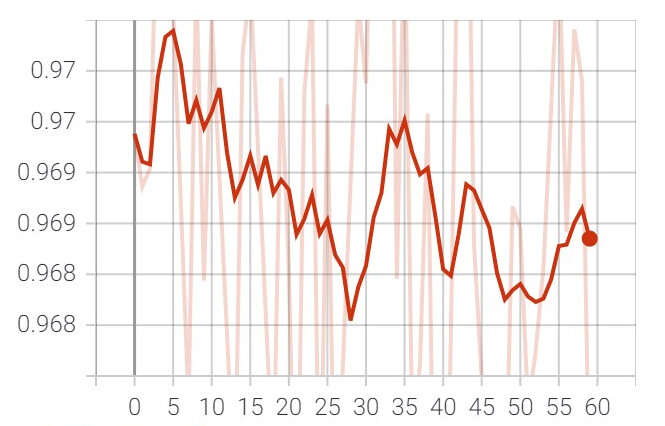# U-Net Segmentation - Dice Loss fluctuating

Hi,

I am trying to build a U-Net Multi-Class Segmentation model for the brain tumor dataset. I implemented the dice loss using nn.module and some guidance from other implementations on the internet. But during my training, my loss is fluctuating and not converging. If I train my model using CrossEntropyLoss it is converging well. When I was debugging with the required_gradient it seems to be False for the output from the loss. I am unable to find out the issue here.

The loss implementation:

``````class DiceLoss(nn.Module):
def __init__(self, weight=None, size_average=True):
super(DiceLoss, self).__init__()
self.weights = weight

def forward(self, inputs, targets, eps=0.001):

inputs = torch.argmax(F.log_softmax(inputs, dim=1), dim=1)
inputs = F.one_hot(inputs, 5).float()
targets = F.one_hot(targets, 5).float()
intersection = (inputs.view(4,-1,5) * targets.view(4,-1,5)).sum(1)
total = (inputs.view(4,-1,5) + targets.view(4,-1,5)).sum(1)
dice = (2 * intersection + eps)/(total + eps)
diceLoss = 1 - (dice.mean(0) * self.weights).sum()/self.weights.sum()

return diceLoss
``````
1 Like

`torch.argmax` is not differentiable and would thus detach the output from the computation graph.
This should also yield an error such as:

``````RuntimeError: element 0 of tensors does not require grad and does not have a grad_fn
``````

so I’m not sure if you are rewrapping the loss in a new tensor or why it’s not raised.
I’m not sure where this implementation comes from, but note that other implementations are using `softmax` to calculate the probabilities for each class.

1 Like

Yes, I got the same error you posted here.

Ok, yeah the argmax seems to detach the node from the graph. Let me try it with F.Softmax.

Thank you!

``````class IoULoss(nn.Module):
def __init__(self, weight=None, size_average=True):
super(IoULoss, self).__init__()
self.weights = weight

def forward(self, inputs, targets, eps=0.001):
inputs = nn.Softmax(dim=1)(inputs)
targets = F.one_hot(targets.long(), 5).float()

intersection = torch.sum((inputs.permute(0,2,3,1)*targets), (1,2))

total = torch.sum((inputs.permute(0,2,3,1) + targets), (1,2))

union = total - intersection

IoU = (intersection + eps)/(union + eps)

iouLoss = 1 - (IoU.mean(0) * self.weights).sum()/self.weights.sum()

return iouLoss
``````

Thank you. It worked now. But unfortunately, the model doesn’t seem to be learning from this loss.1 Like